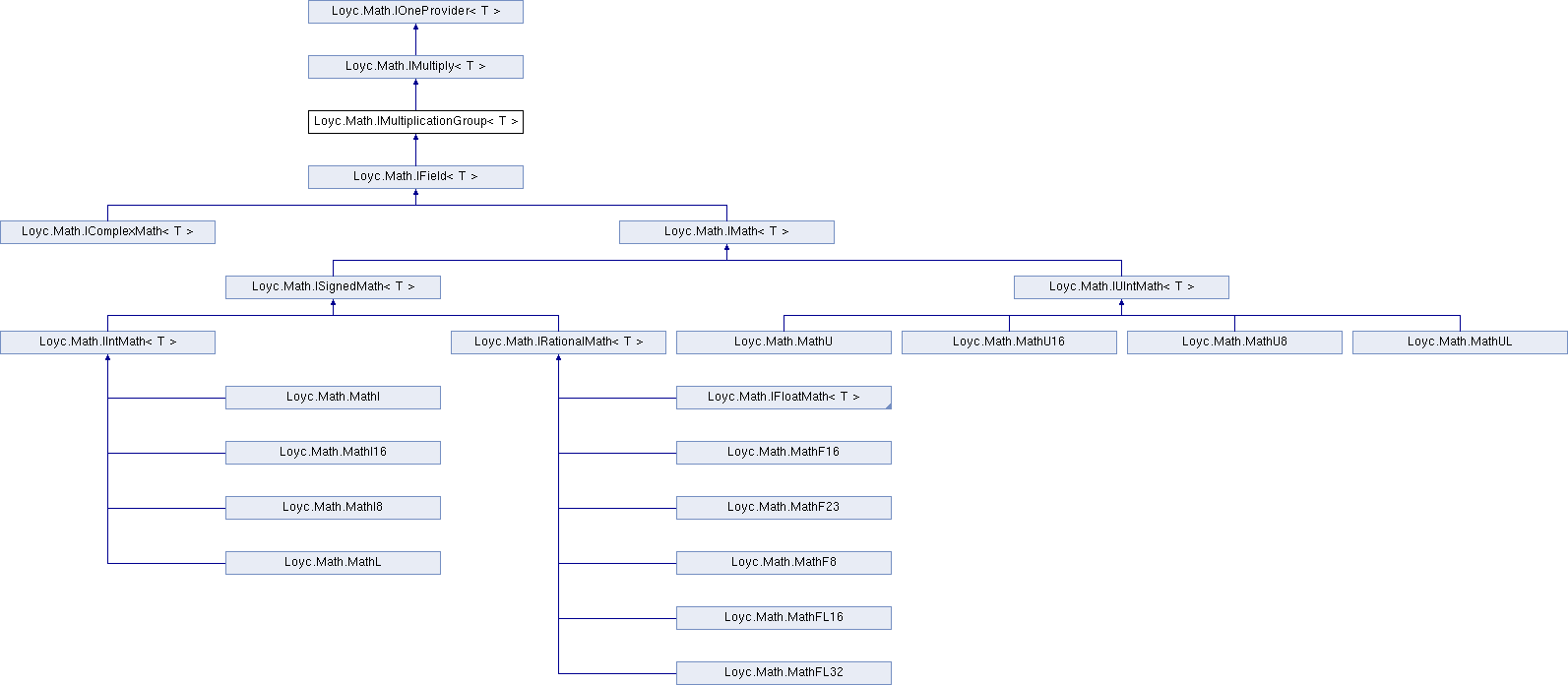Enhanced C# Language of your choice: library documentation

# Documentation moved to ecsharp.net

GitHub doesn't support HTTP redirects, so you'll be redirected in 3 seconds.

Loyc.Math.IMultiplicationGroup< T > Interface Template Reference

This defines a Group with the operation *, the neutral element One, the inverse Inverse and an operation / that is defined in terms of the inverse. More...

Source file:
Inheritance diagram for Loyc.Math.IMultiplicationGroup< T >:## Remarks

This defines a Group with the operation *, the neutral element One, the inverse Inverse and an operation / that is defined in terms of the inverse.

Axioms that have to be satisified by the operations: Commutativity of multiplication: Multiply(a,b)=Multiply(b,a) for all a,b in T Associativity of multiplication: Multiply(Multiply(a,b),c)=Multiply(a,Multiply(b,c)) Inverse of multiplication: Multiply(a,Inverse(a))==One for all a in T Divison: Divide(a,b)==Multiply(a,Inverse(b)) for all a in T Neutral element: Multiply(One,a)==a for all a in T

ShiftLeft and ShiftRight operations are commonly thought of as binary operations, but some algorithms need to multiply numbers by powers of two and want to do so efficiently, while still supporting floating-point types. Therefore it makes sense to offer ShiftLeft ("multiply by a power of two") and ShiftRight ("divide by a power of two") operators as part of the multiple/divide interface, not just IBinaryMath{T}. Even floating-point types can support these two operations efficiently by directly modifying the exponent part of the floating-point representation.

## Public Member Functions

Div (T a, T b)

Shl (T a, int amount)

Shr (T a, int amount)

MulDiv (T a, T mulBy, T divBy)Public Member Functions inherited from Loyc.Math.IMultiply< T >
Mul (T a, T b)Properties inherited from Loyc.Math.IOneProvider< T >
One` [get]`